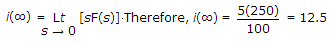# Electronics and Communication Engineering - Signals and Systems

Exercise : Signals and Systems - Section 1
26.
A function having frequency f is to be sampled. The sampling time T should be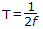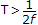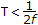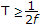Explanation:

Sampling frequency must be more than 2f. Therefore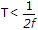.

27.
The final value theorem is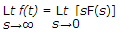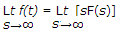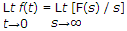Explanation:

£f(t) =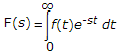£-1F(s) = f(t)

£[a f1(t) + bf2(t)] = aF1(s) + bF2(s)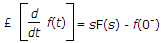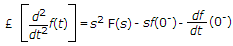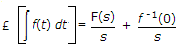where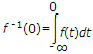£[f(t - T)] = e-sT F(s)

£[e-at f(t)] = F(s + a)

Initial value theorem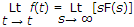Final value theroem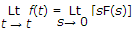Convolution Integral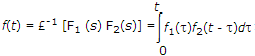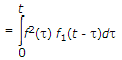where t is dummy variable for t.

28.
If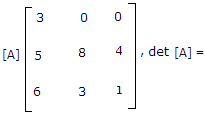- 12
12
20
- 20
Explanation:

det.|A| = 3(8 - 12) = - 12.

29.
Inverse Fourier transform of sgn (ω) is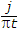1
U(t)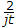Explanation:

By duality Property.

30.
If I (s)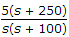, the final value of i(t) is
5A
12.5 A
0.05 A
1250 A